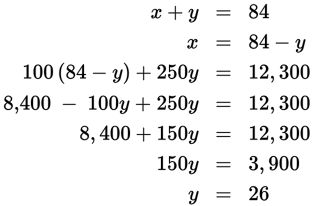# SAT Math Multiple Choice Question 808: Answer and Explanation

### Test Information

Question: 808

13. A small office supply store sells paper clips in packs of 100 and packs of 250. Ifthe store has 84 packs of paper clips in stock totaling 12,300 paper clips, how manypaper clips would a customer buy if he buys half of the packs of 250 that the storehas in stock?

• A. 2,900
• B. 3,250
• C. 5,800
• D. 6,500

Explanation:

B

Difficulty: Hard

Category: Heart of Algebra / Systems of Linear Equations

Strategic Advice: Create a system of linear equations where x represents the number of packs with 100 paper clips and y represents the number of packs with 250 paper clips. Before selecting your final answer, make sure you are answering the right question (how many paper clips a customer would buy if he buys half of the packs of 250).

Getting to the Answer: The first equation should represent the total number of packs, each with 100 or 250 paper clips, or x + y = 84. The second equation should represent the total number of paper clips. Because x represents packs with 100 paper clips and y represents packs with 250 paper clips, the second equation is 100x + 250y = 12,300. Now solve the system using substitution. Solve the first equation for either variable, and substitute the result into the second equation:The store has 26 packs of 250 paper clips in stock. The question asks about packs of 250, so you don't need to find the value of x. You aren't done yet, however. The problem asks how many paper clips a customer would buy if he buys half of the packs of 250 the store has. The customer would buy 13 × 250 = 3,250 paper clips, which is (B).